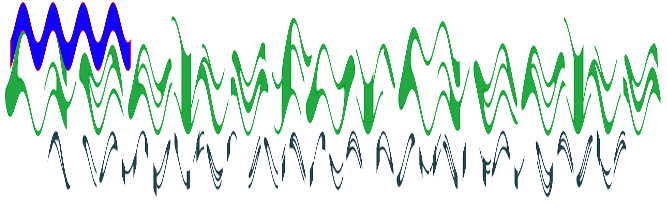# Node.js GM wave() Function

• Last Updated : 13 Oct, 2021

The wave() function is an inbuilt function in the GraphicsMagick library which is used to alter an image along with a sine wave. The function returns the true value on success.

Syntax:

`wave( amplitude, wavelength )`

Parameters: This function accept two parameters as mentioned above and described below:

• amplitude: This parameter stores the value of the amplitude of the sine wave.
• wavelength: This parameter stores the value of the wavelength of the sine wave.

Return Value: This function returns GraphicsMagick object with image added.

Original Image:Example 1:

 `// Include gm library``var` `gm = require(``'gm'``);`` ` `// Import the image``gm(``'gfg.png'``)`` ` `// Invoke wave function with an amplitude``// values as 10 and wavelength as 3``.wave(10, 3)`` ` `// Process and Write the image``.write(``"wave1.png"``, ``function` `(err) {``  ``if` `(!err) console.log(``'done'``);``});`

Output:Example 2:

 `// Include gm library``var` `gm = require(``'gm'``);``  ` `// Import the image``gm(``'gfg.png'``)``  ` `// Set stroke color``.stroke(``"#fe1232"``)``  ` `// Set fill color``.fill(``"#1200ff"``)``  ` `// Draw Rectangle using drawRectangle function``.drawRectangle(10, 2, 130, 30, 1, 2)``  ` `// Invoke wave function with an amplitude``// as 20 and wavelength as 30``.wave(20, 30)``  ` `// Process and Write the image``.write(``"wave2.png"``, ``function` `(err) {``    ``if` `(!err) console.log(``'done'``);``});`

Output:Reference:

My Personal Notes arrow_drop_up